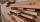# Volume + third power - math problems

#### Number of problems found: 72

• Cylinder - areaThe diameter of the cylinder is one-third the length of the height of the cylinder. Calculate the surface of cylinder if its volume is 2 m3.
• Volume of ballFind the volume of a volleyball that has a radius of 4 1/2 decimeters. Use 22/7 for π
• The volume 2The volume of a cube is 27 cubic meters. Find the height of the cube.
• Sphere fallHow many percent fall volume of a sphere if diameter decrease 3×?
• Sphere growthHow many times grow volume of sphere if diameter rises 10×?
• Cube in ballCube is inscribed into the sphere of radius 181 dm. How many percent is the volume of cube of the volume of the sphere?
• Cube 3How many times will increase the volume of a cube if we double the length of its edge?
• Cube surfce2volumeCalculate the volume of the cube if its surface is 150 cm2.
• Volume and areaWhat is the volume of a cube which has area of 361 cm2?
• Cube basicsHow long is the edge length of a cube with volume 15 m3?
• Cube edgesIf the edge length of the cube increases by 50%, how does the volume of this cube increase?
• CubeThe sum of lengths of cube edges is 57 cm. What is its surface and volume?
• Volume and body diagonalCalculate how much the volume and body diagonal of the cuboid decrease if we reduce each of its three edges a, b, c by 18%?
• CubeOne cube has edge increased 5 times. How many times will larger its surface area and volume?
• Density 2What is the density of a piece of driftwood that has the mass of 25 grams and a volume is 30 cm to the 3rd power. And how do I figure it out?
• Surface of the cylinderCalculate the surface area of the cylinder when its volume is 45 l and the perimeter of base is three times of the height.
• Cube diagonalsCalculate the length of the side and the diagonals of the cube with a volume of 27 cm3.
• LatheCalculate the percentage of waste if the cube with 53 cm long edge is lathed to cylinder with a maximum volume.
• Cube V2SThe volume of the cube is 27 dm cubic. Calculate the surface of the cube.
• Edges or sidesCalculate the cube volume, if the sum of the lengths of all sides is 276 cm.

Do you have an interesting mathematical word problem that you can't solve it? Submit a math problem, and we can try to solve it.

We will send a solution to your e-mail address. Solved examples are also published here. Please enter the e-mail correctly and check whether you don't have a full mailbox.

Please do not submit problems from current active competitions such as Mathematical Olympiad, correspondence seminars etc...

Tip: Our volume units converter will help you with the conversion of volume units. Volume - math word problems. Third power - math word problems.